# RD Sharma Solutions for Class 11 Maths Exercise 21.1 Chapter 21 - Some Special Series

In Exercise 21.1 of Chapter 21, we shall discuss problems based on the sum of the first ‘n’ natural numbers, the sum of the squares of first ‘n’ natural numbers, the sum of the cubes of first ‘n’ natural numbers. Students wishing to clear their doubts pertaining to this exercise can utilise the RD Sharma Class 11 Maths Solutions. The solutions are formulated by our expert faculty team in a comprehensive manner to make it interesting for students to solve. The links to the solutions of this exercise can be accessed in the RD Sharma Class 11 Maths pdf, which is available in the below-mentioned links.

## Download the pdf of RD Sharma Solutions for Class 11 Maths Exercise 21.1 Chapter 21 – Some Special Series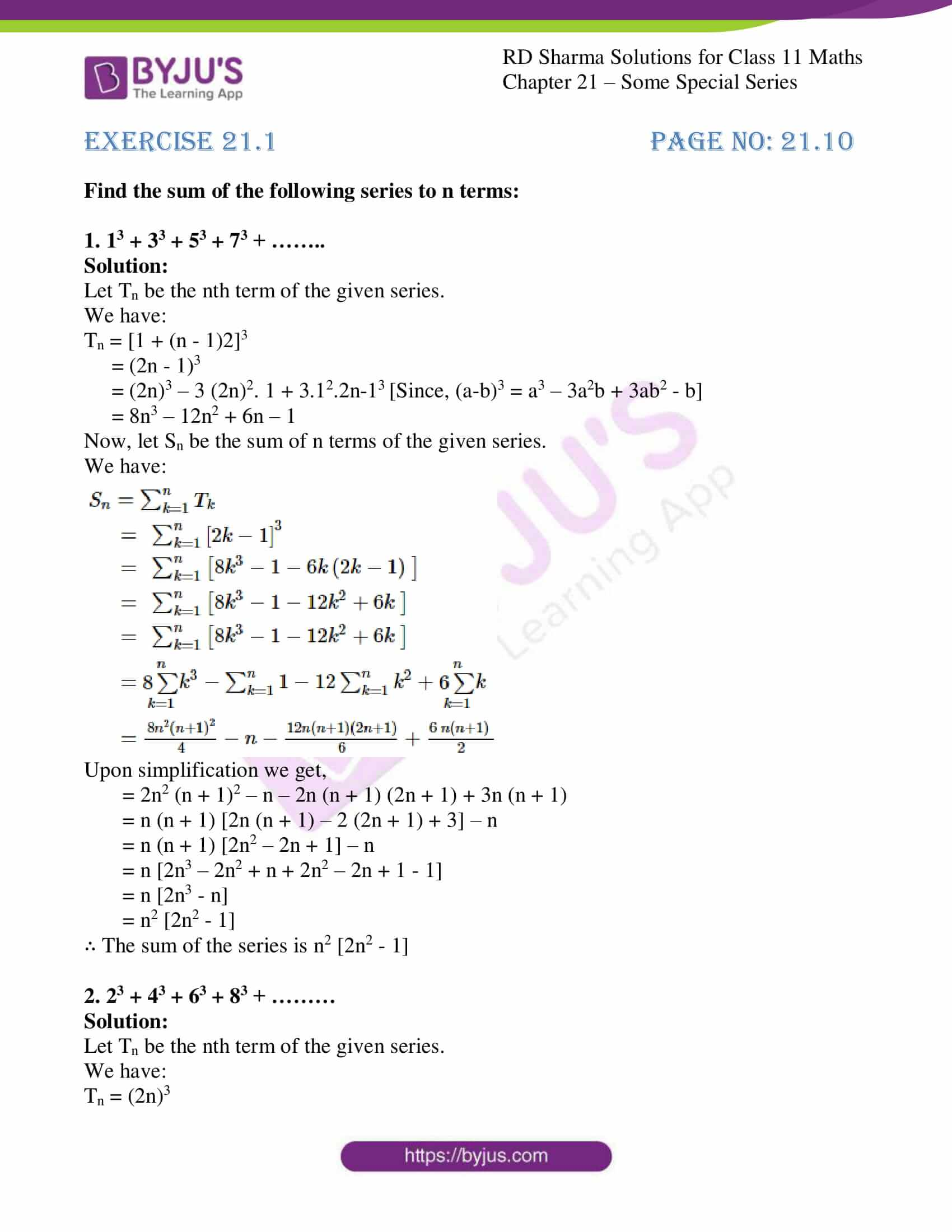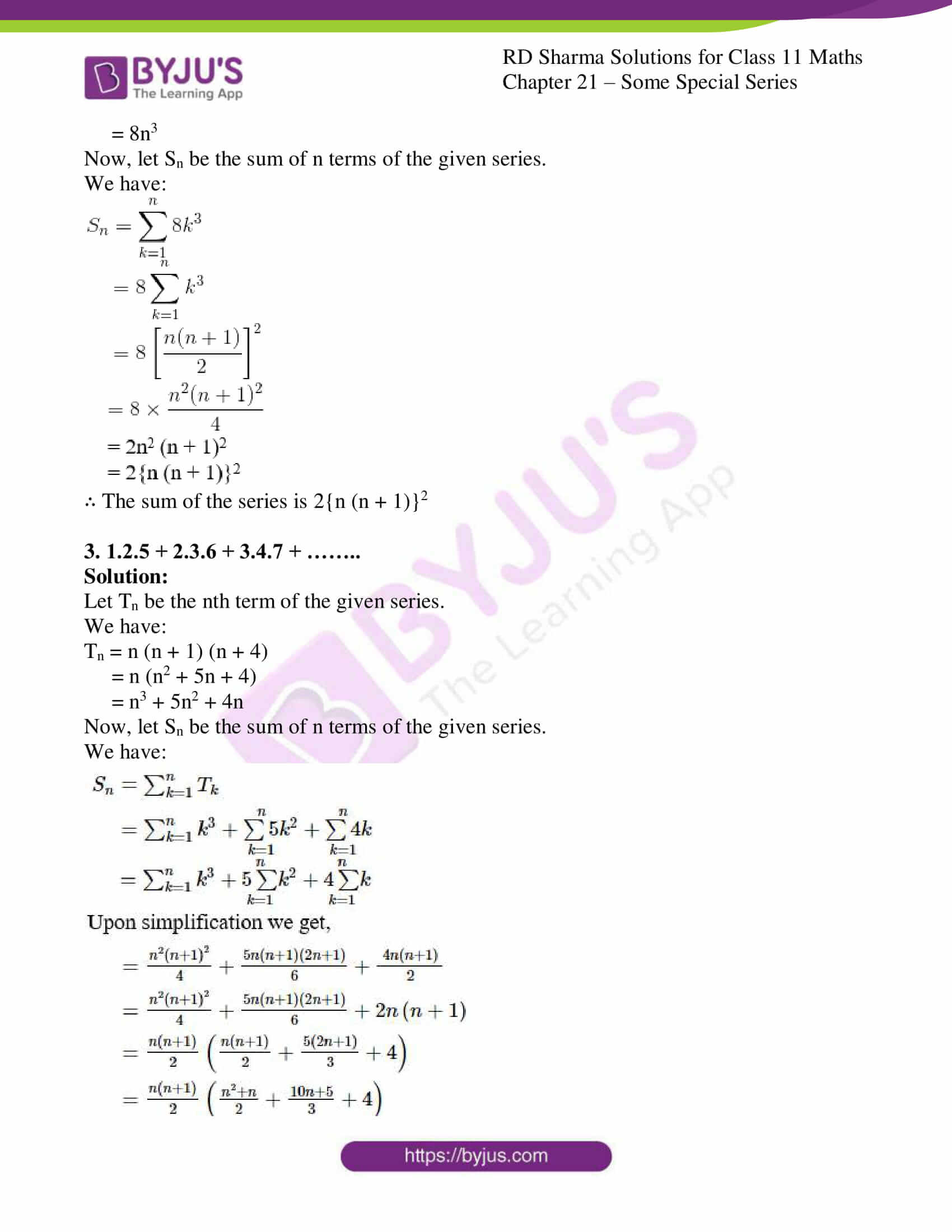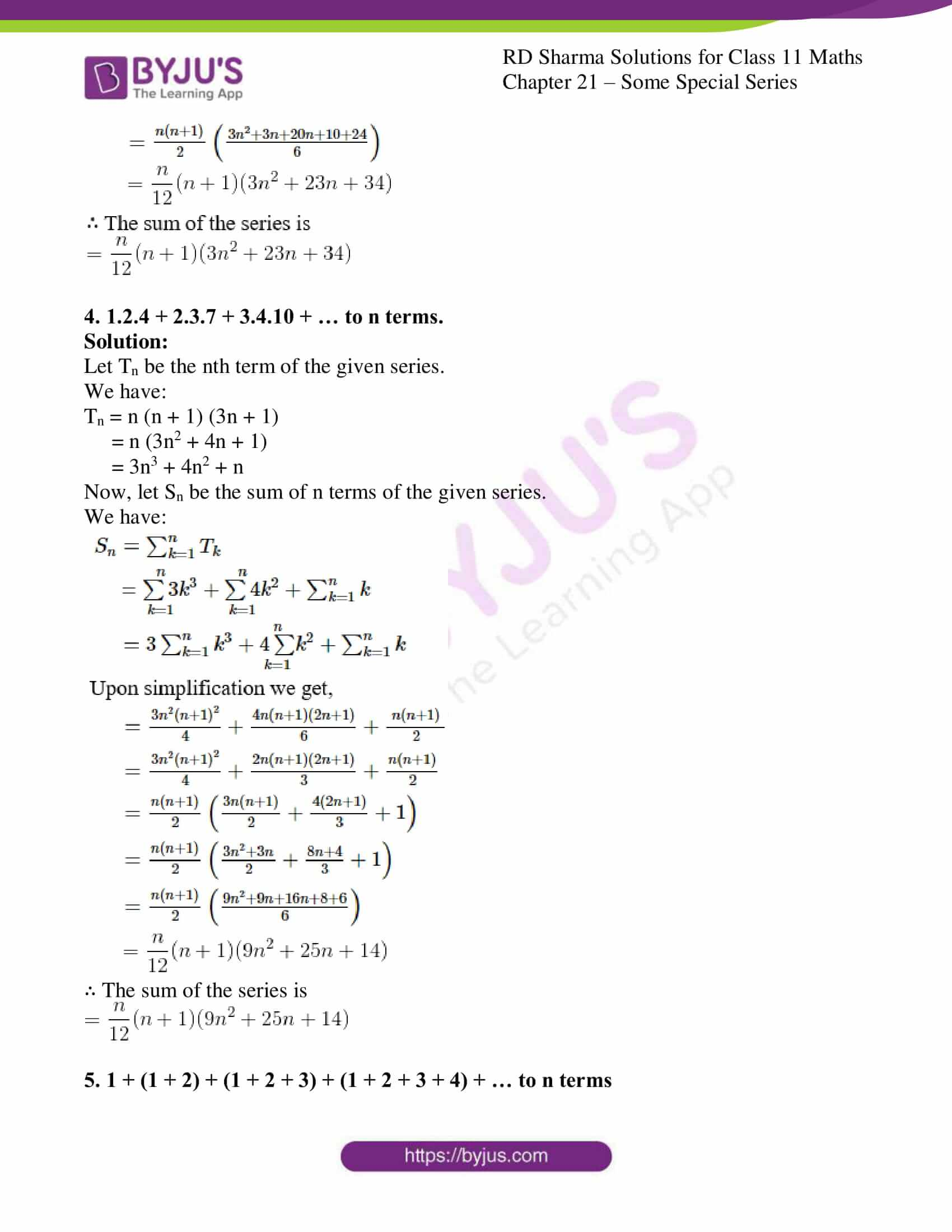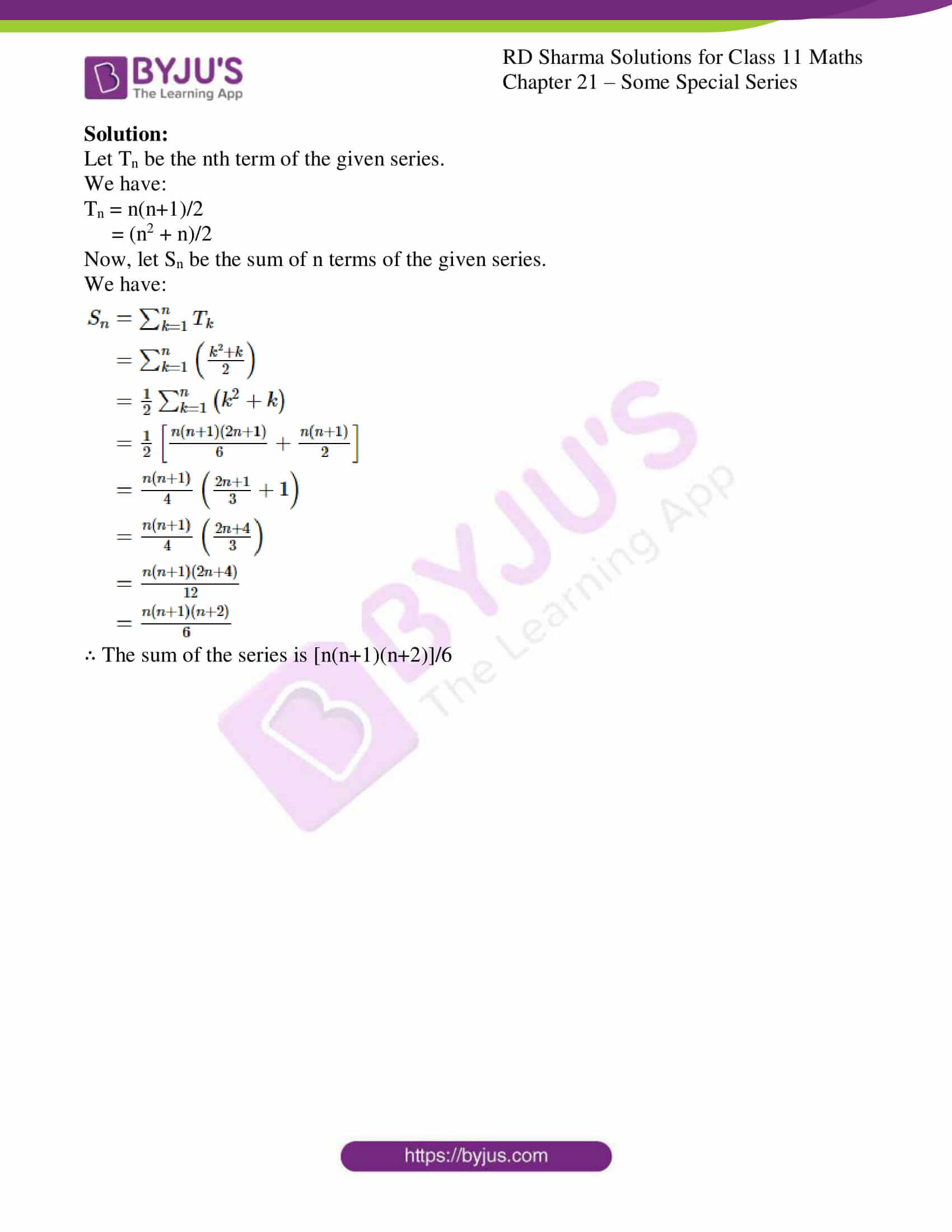### Access answers to RD Sharma Solutions for Class 11 Maths Exercise 21.1 Chapter 21 – Some Special Series

Find the sum of the following series to n terms:

1. 13 + 33 + 53 + 73 + ……..

Solution:

Let Tn be the nth term of the given series.

We have:

Tn = [1 + (n – 1)2]3

= (2n – 1)3

= (2n)3 – 3 (2n)2. 1 + 3.12.2n-13 [Since, (a-b)3 = a3 – 3a2b + 3ab2 – b]

= 8n3 – 12n2 + 6n – 1

Now, let Sn be the sum of n terms of the given series.

We have: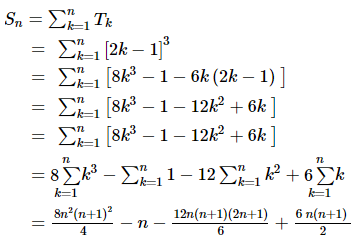Upon simplification we get,

= 2n2 (n + 1)2 – n – 2n (n + 1) (2n + 1) + 3n (n + 1)

= n (n + 1) [2n (n + 1) – 2 (2n + 1) + 3] – n

= n (n + 1) [2n2 – 2n + 1] – n

= n [2n3 – 2n2 + n + 2n2 – 2n + 1 – 1]

= n [2n3 – n]

= n2 [2n2 – 1]

∴ The sum of the series is n2 [2n2 – 1]

2. 23 + 43 + 63 + 83 + ………

Solution:

Let Tn be the nth term of the given series.

We have:

Tn = (2n)3

= 8n3

Now, let Sn be the sum of n terms of the given series.

We have: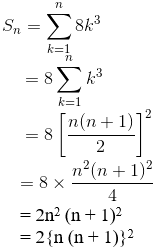∴ The sum of the series is 2{n (n + 1)}2

3. 1.2.5 + 2.3.6 + 3.4.7 + ……..

Solution:

Let Tn be the nth term of the given series.

We have:

Tn = n (n + 1) (n + 4)

= n (n2 + 5n + 4)

= n3 + 5n2 + 4n

Now, let Sn be the sum of n terms of the given series.

We have: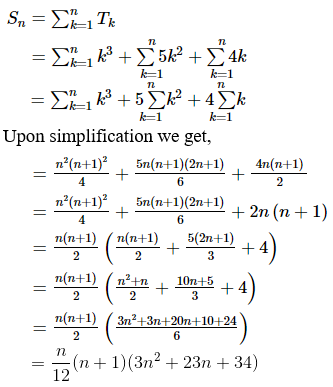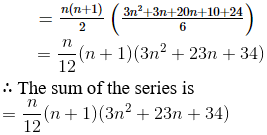4. 1.2.4 + 2.3.7 + 3.4.10 + … to n terms.

Solution:

Let Tn be the nth term of the given series.

We have:

Tn = n (n + 1) (3n + 1)

= n (3n2 + 4n + 1)

= 3n3 + 4n2 + n

Now, let Sn be the sum of n terms of the given series.

We have: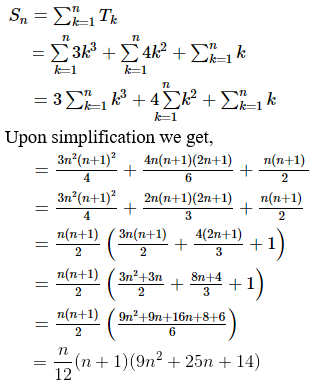∴ The sum of the series is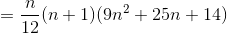5. 1 + (1 + 2) + (1 + 2 + 3) + (1 + 2 + 3 + 4) + … to n terms

Solution:

Let Tn be the nth term of the given series.

We have:

Tn = n(n+1)/2

= (n2 + n)/2

Now, let Sn be the sum of n terms of the given series.

We have: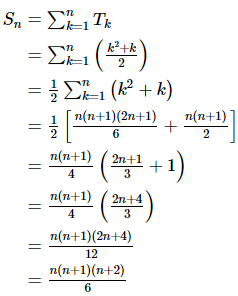∴ The sum of the series is [n(n+1)(n+2)]/6